Next: Eigenvalues of Orbital Angular Up: Orbital Angular Momentum Previous: Orbital Angular Momentum

# Orbital Angular Momentum

Consider a particle described by the Cartesian coordinates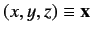and their conjugate momenta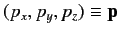. The classical definition of the orbital angular momentum of such a particle about the origin is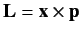, giving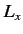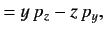(290)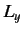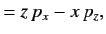(291)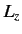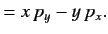(292)

Let us assume that the operators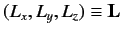which represent the components of orbital angular momentum in quantum mechanics can be defined in an analogous manner to the corresponding components of classical angular momentum. In other words, we are going to assume that the above equations specify the angular momentum operators in terms of the position and linear momentum operators. Note that,, andare Hermitian, so they represent things which can, in principle, be measured. Note, also, that there is no ambiguity regarding the order in which operators appear in products on the right-hand sides of Equations (290)-(292), because all of the products consist of operators that commute.

The fundamental commutation relations satisfied by the position and linear momentum operators are [see Equations (114)-(116)]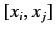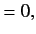(293)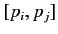(294)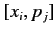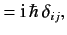(295)

where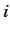and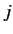stand for either,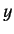, or. Consider the commutator of the operatorsand: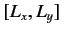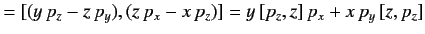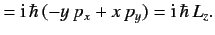(296)

The cyclic permutations of the above result yield the fundamental commutation relations satisfied by the components of an orbital angular momentum: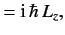(297)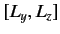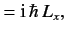(298)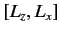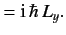(299)

These can be summed up more succinctly by writing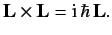(300)

The three commutation relations (297)-(299) are the foundation for the whole theory of angular momentum in quantum mechanics. Whenever we encounter three operators having these commutation relations, we know that the dynamical variables that they represent have identical properties to those of the components of an angular momentum (which we are about to derive). In fact, we shall assume that any three operators that satisfy the commutation relations (297)-(299) represent the components of some sort of angular momentum.

Suppose that there areparticles in the system, with angular momentum vectors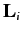(whereruns from 1 to). Each of these vectors satisfies Equation (300), so that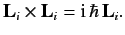(301)

However, we expect the angular momentum operators belonging to different particles to commute, because they represent different degrees of freedom of the system. So, we can write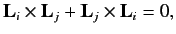(302)

for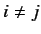. Consider the total angular momentum of the system,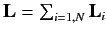. It is clear from Equations (301) and (302) that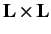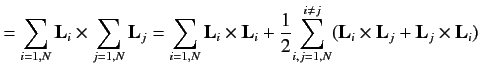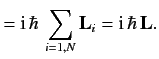(303)

Thus, the sum of two or more angular momentum vectors satisfies the same commutation relation as a primitive angular momentum vector. In particular, the total angular momentum of the system satisfies the commutation relation (300).

The immediate conclusion which can be drawn from the commutation relations (297)-(299) is that the three components of an angular momentum vector cannot be specified (or measured) simultaneously. In fact, once we have specified one component, the values of other two components become uncertain. It is conventional to specify the-component,.

Consider the magnitude squared of the angular momentum vector,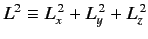. The commutator ofandis written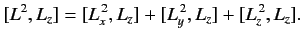(304)

It is easily demonstrated that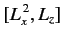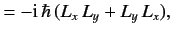(305)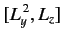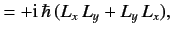(306)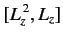(307)

so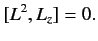(308)

Because there is nothing special about the-direction, we conclude thatalso commutes withand. It is clear from Equations (297)-(299) and (308) that the best we can do in quantum mechanics is to specify the magnitude of an angular momentum vector along with one of its components (by convention, the-component).

It is convenient to define the shift operators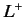and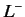: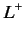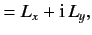(309)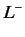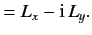(310)

It can easily be shown that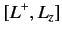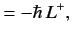(311)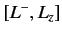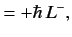(312)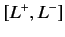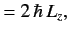(313)

and also that both shift operators commute with.Next: Eigenvalues of Orbital Angular Up: Orbital Angular Momentum Previous: Orbital Angular Momentum
Richard Fitzpatrick 2013-04-08